## 6.3.3 3D Vertical Decomposition

It turns out that the vertical decomposition method of Section 6.2.2 can be extended to any dimension by recursively applying the sweeping idea. The method requires, however, that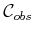is piecewise linear. In other words,is represented as a semi-algebraic model for which all primitives are linear. Unfortunately, most of the general motion planning problems involve nonlinear algebraic primitives because of the nonlinear transformations that arise from rotations. Recall the complicated algebraicmodel constructed in Section 4.3.3. To handle generic algebraic models, powerful techniques from computational algebraic geometry are needed. This will be covered in Section 6.4.

One problem for whichis piecewise linear is a polyhedral robot that can translate in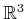, and the obstacles in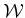are polyhedra. Since the transformation equations are linear in this case,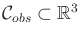is polyhedral. The polygonal faces ofare obtained by forming geometric primitives for each of the Type FV, Type VF, and Type EE cases of contact between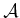and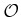, as mentioned in Section 4.3.2.

Figure 6.20 illustrates the algorithm that constructs the 3D vertical decomposition. Compare this to the algorithm in Section 6.2.2. Let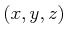denote a point in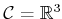. The vertical decomposition yields convex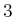-cells,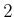-cells, and-cells. Neglecting degeneracies, a generic-cell is bounded by six planes. The cross section of a-cell for some fixedvalue yields a trapezoid or triangle, exactly as in the 2D case, but in a plane parallel to the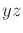plane. Two sides of a generic-cell are parallel to theplane, and two other sides are parallel to the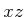plane. The 3-cell is bounded above and below by two polygonal faces of.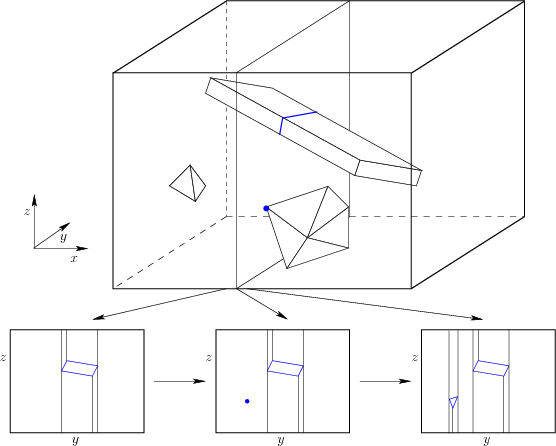Initially, sort thevertices by theircoordinate to obtain the events. Now consider sweeping a plane perpendicular to the-axis. The plane for a fixed value ofproduces a 2D polygonal slice of. Three such slices are shown at the bottom of Figure 6.20. Each slice is parallel to theplane and appears to look exactly like a problem that can be solved by the 2D vertical decomposition method. The-cells in a slice are actually slices of-cells in the 3D decomposition. The only places in which these-cells can critically change is when the sweeping plane stops at somevalue. The center slice in Figure 6.20 corresponds to the case in which a vertex of a convex polyhedron is encountered, and all of the polyhedron lies to right of the sweep plane (i.e., the rest of the polyhedron has not been encountered yet). This corresponds to a place where a critical change must occur in the slices. These are 3D versions of the cases in Figure 6.2, which indicate how the vertical decomposition needs to be updated. The algorithm proceeds by first building the 2D vertical decomposition at the firstevent. At each event, the 2D vertical decomposition must be updated to take into account the critical changes. During this process, the 3D cell decomposition and roadmap can be incrementally constructed, as in the 2D case.

The roadmap is constructed by placing a sample point in the center of each-cell and-cell. The vertices are the sample points, and edges are added to the roadmap by connecting the sample points for each case in which a-cell is adjacent to a-cell.

This same principle can be extended to any dimension, but the applications to motion planning are limited because the method requires linear models (or at least it is very challenging to adapt to nonlinear models; in some special cases, this can be done). See  for a summary of the complexity of vertical decompositions for various geometric primitives and dimensions.

Steven M LaValle 2020-08-14